# 圆的反演变换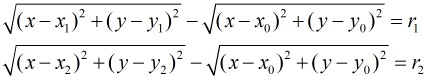(1)除反演中心外，平面上的每一个点都只有唯一的反演点，且这种关系是对称的，位于反演圆上的点，保持在原处，位于

(2)任意一条不过反演中心的直线，它的反形是经过反演中心的圆，反之亦然，特别地，过反演中心相交的圆，变为不过反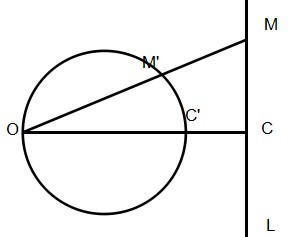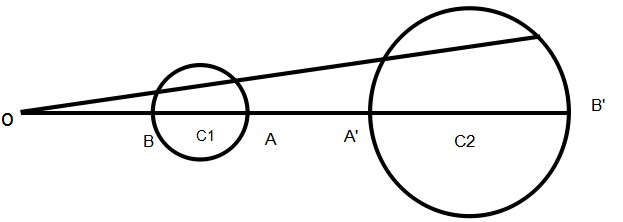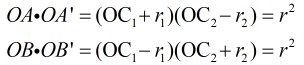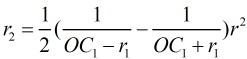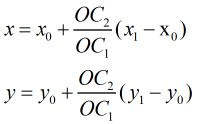#include <iostream>
#include <string.h>
#include <stdio.h>
#include <math.h>

using namespace std;
double const eps = 1e-8;

struct Point
{
double x,y;
Point(double a = 1.0,double b = 1.0):x(a),y(b){}
Point operator + (const Point &a)
{
return Point(x+a.x,y+a.y);
}
Point operator - (const Point &a)
{
return Point(x-a.x,y-a.y);
}
Point operator * (const double a)
{
return Point(a*x,a*y);
}
Point Trans()
{
return Point(-y,x);
}
void Input()
{
scanf("%lf%lf",&x,&y);
}
} ;

struct Circle
{
Point o;
double r;
Circle(Point a = Point(),double b = 1.0):o(a),r(b) {}
Point getPoint(double alpha)
{
return o + Point(r*cos(alpha),r*sin(alpha));
}
void Input()
{
o.Input();
scanf("%lf",&r);
}
void Output()
{
printf("%.8lf %.8lf %.8lf\n",o.x,o.y,r);
}
} ;

Point p;
Circle c;

double dist(Point A,Point B)
{
return sqrt((A.x-B.x)*(A.x-B.x) + (A.y-B.y)*(A.y-B.y));
}

double cross(Point A,Point B,Point C)
{
return (B.x-A.x)*(C.y-A.y) - (B.y-A.y)*(C.x-A.x);
}

int sign(double x)
{
return (x > eps) - (x < -eps);
}

Circle Inverse(Circle C)
{
Circle T;
double t = dist(C.o,p);
double x = 1.0 / (t - C.r);
double y = 1.0 / (t + C.r);
T.r = (x - y) / 2.0;
double s = (x + y) / 2.0;
T.o = p + (C.o - p) * (s / t);
return T;
}

{
double t = cross(a,p,b);
if(t < 0) t = -t;
double d = dist(a,b);
t /= d;
if(t > eps)
{
double w = 0.5 / t;
Point dir = (b-a).Trans();
Point a1 = p + dir * (w / d);
Point b1 = p - dir * (w / d);
if(fabs(cross(a,b,a1)) < fabs(cross(a,b,b1)))
c[k++] = Circle(a1,w);
else
c[k++] = Circle(b1,w);
}
}

int Work()
{
c = Inverse(c);
c = Inverse(c);
if(c.r > c.r) swap(c,c);
Point v = c.o - c.o;
double alpha = atan2(v.y,v.x);
double d = dist(c.o,c.o);
double beta  = acos((c.r - c.r) / d);
int k = 2;
Point a = c.getPoint(alpha + beta);
Point b = c.getPoint(alpha + beta);
if(sign(cross(a,c.o,b)) == sign(cross(a,p,b)) &&
sign(cross(a,c.o,b)) == sign(cross(a,p,b)))
a = c.getPoint(alpha - beta);
b = c.getPoint(alpha - beta);
if(sign(cross(a,c.o,b)) == sign(cross(a,p,b)) &&
sign(cross(a,c.o,b)) == sign(cross(a,p,b)))
return k - 2;
}

int main()
{
int T;
scanf("%d",&T);
while(T--)
{
c.Input();
c.Input();
p.Input();
int num = Work();
printf("%d\n",num);
for(int i=0;i<num;i++)
c[i+2].Output();
}
return 0;
}


10-30
09-1235
12-28183
11-231668
02-05128
02-2471
09-17
08-122709
04-03
06-26756
01-22710
02-28432
08-221309
07-25569
08-12424

### “相关推荐”对你有帮助么？

•非常没帮助
•没帮助
•一般
•有帮助
•非常有帮助被折叠的  条评论 为什么被折叠?到【灌水乐园】发言ACdreamers

¥2 ¥4 ¥6 ¥10 ¥20余额支付 (余额：-- )扫码支付获取中扫码支付点击重新获取扫码支付1.余额是钱包充值的虚拟货币，按照1:1的比例进行支付金额的抵扣。
2.余额无法直接购买下载，可以购买VIP、C币套餐、付费专栏及课程。余额充值